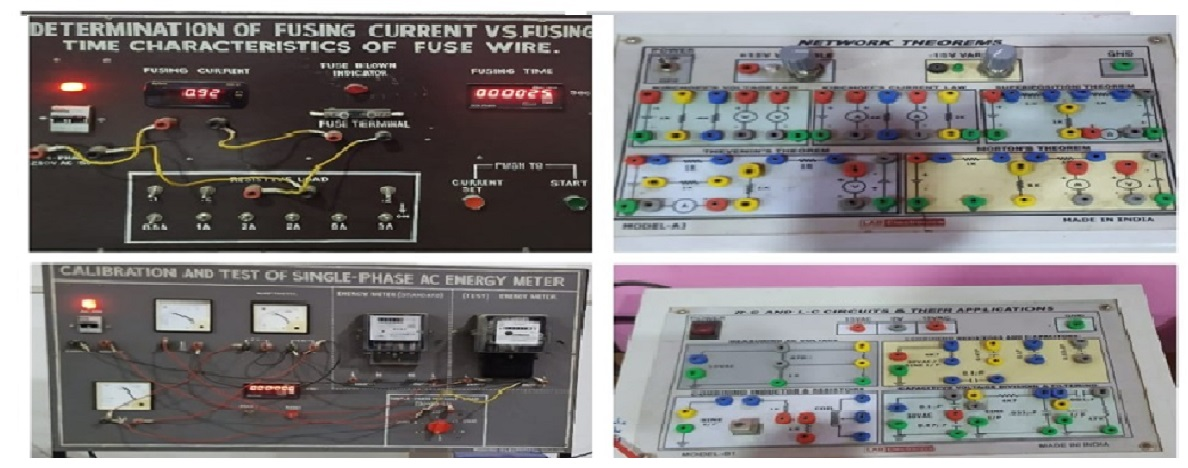## Basic Electrical Engineering Laboratory

Basic Electrical Enineering Laboratory is used with institute core course "Introduction to Electrical Engineering". This lab can accommodate 85 students in a semester. In this lab students of first year perform experiments related to topics taught in theory like Kirchhoff’s current and voltage laws, Norton’s theorem, thevenin’s theorem, RLC circuits , energy meters, fuse characteristics, open circuit and short circuit test of single phase transformers, etc. The Experiments are designed to expose students to the practical implementation of the fundamental theories of Electrical Engineering.

## Utilization

The Basic Electrical Engineering Laboratory is utilized for performing experiments related to fundamentals of Electrical circuit and machine devices by all first year undergraduate students of the college.## Equipments

Sl. no. Equipments
1. Setup to test Fusing Characteristics.
2. Setup to test Energy meter
3. Setup to verify Network Theorems
4. Variable power supply
5. Measuring insulation resistance by Megger
6. Study of three phase Transformer connections
7. Digital CROs
8. P-N Diode Channel Trainer
9. Network  Theorem Trainer
10. R-L-C Circuit Trainer Kit